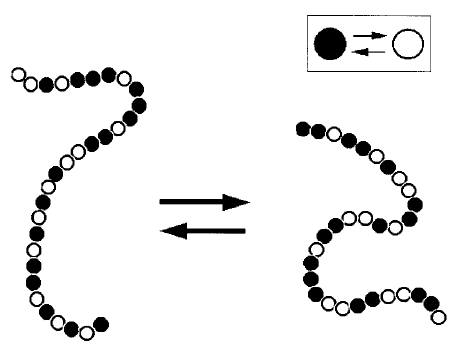# Concentration dependence of the Flory (X) parameter within two-state models`[button href="http://pubs.acs.org/doi/pdf/10.1021/ma020296o" color="lime_green" target="_blank" id=""]PDF[/button]`
`[button href="http://arxiv.org/pdf/cond-mat/0202264v2" color="sea_foam" target="_blank" id=""]arXiv[/button]`

### V. A. Baulin and A. Halperin

##### Macromolecules, 35, 6432 – 6438 (2002)

The Flory χ parameter is typically assumed to depend only on the temperature, T. Experimental results often require the replacement of this χ(T) by χeff, which depends also on the monomer volume fraction, φ, χeff(φ,T). Such χeff(φ,T) can arise from two-state models, proposed for polyetheleneoxide (PEO) and other neutral water-soluble polymers. The predicted φ dependence of χ = χeff − (1 − φ)∂χeff/∂φ, obtainable from colligative properties, differs qualitatively between the various models:  (i) The model of Karlstrom (J. Phys. Chem.198589, 4962) yields ∂χ/∂φ ≥ 0 while the model of Matsuyama and Tanaka (Phys. Rev. Lett.199065, 341) and of Bekiranov et al. (Phys. Rev. E199755, 577) allows for ∂χ/∂φ < 0. (ii) χ(φ) as calculated from the Karlstrom model, utilizing the parameters used to fit the phase diagram of PEO, agrees semiquantitatively with the experimental values. On the other hand, χ(φ) similarly calculated from the model of Bekiranov et al. differs qualitatively from the measured results. Altogether, χ(φ) provides useful measure for the performance of a model.

[people_short
url=”https://softmat.net/t2t_products/baulin/”
]

5
0%
4
0%
3
0%
2
0%
1
0%
0
0%